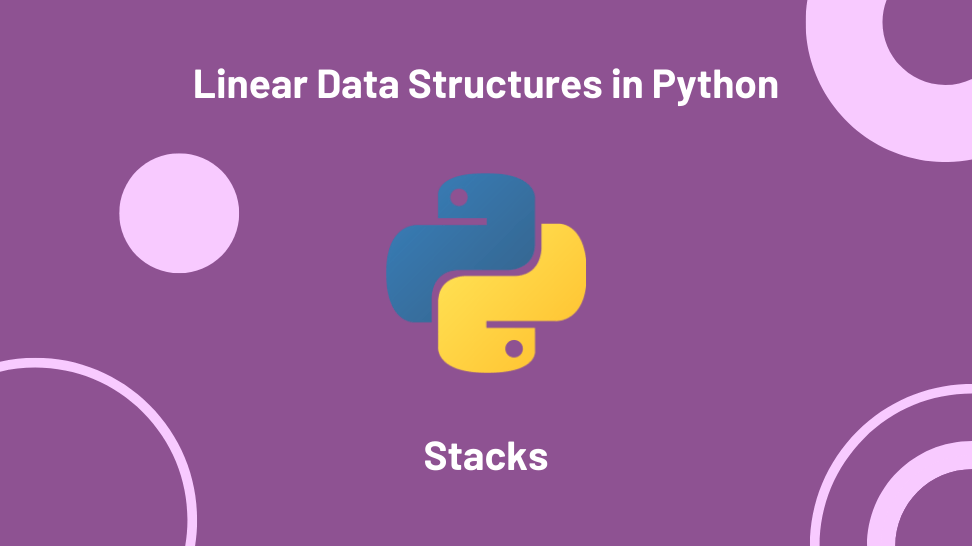In this article, I’ll explore the stack, a linear data structure we can easily implement in Python.

This post is the first in a miniseries exploring the implementation of linear data structures. I based the series on my notes while studying for a Python technical interview.

For quick access, here is the list of posts on this series:

2. A Queue Implementation in Python
3. A Priority Queue Implementation in Python
4. Linked Lists Implementations In Python

## Introduction to stacks

In computer science, a stack is an abstract data type serving as a collection of elements. The stack has two primary operations:

1. Push, which adds an element to the collection
2. Pop, which removes the most recently added element still in the collection

You add and remove elements from a stack in a last-in, first-out fashion (LIFO).

## Uses for stacks

In programming, you can use stacks to implement applications and functionality that require LIFO data access. Some examples might be:

1. The undo functionality in your text editor.
2. The back button in your browser.
3. The call stack in your favorite programming language.

## Stack methods

Most stack implementations have the following methods:

1. stack.push(x): adds an element to the top of the stack.
2. stack.pop(): returns and removes the element at the top of the stack.
3. stack.peek(): returns the element at the top of the stack.
4. stack.size(): returns the number of elements in the stack.
5. stack.is_empty(): returns True is the stack is empty.

## Stack implementation

For our stack implementation, we will use the double-ended queue (deque) available from the collections module in Python. The reason for using deques instead of other data types like lists are:

1. In deques, appends and pops are memory efficient and thread-safe.
2. In deques, appends and pops have constant time complexity O(1).

Let’s go over our stack implementation using deques.

### The Stack class

We define a Stack class with a constructor that instantiates an empty deque and a __str__ method that returns a readable representation of the stack object. At the top of the module, we must import deque from collections:

### stack.is_empty():

The is_empty method returns True if the stack is empty.

This method has a constant time complexity O(1).

### stack.push(item)

The push method adds an item to the stack, using the append method from deque.

This method has a constant time complexity O(1).

### stack.pop()

The pop method returns and removes the item at the top of the stack, using the pop method from deque. To avoid getting an IndexError when popping from an empty stack, we first check if it is empty and return None.

This method has a constant time complexity O(1).

### stack.peek()

The peek method returns the item at the top of the stack by slicing. As with pop, we need to return None if the stack is empty to avoid an IndexError.

This method has a constant time complexity O(1).

### stack.size()

The size method returns the length of the stack.

This method has a constant time complexity O(1).

### The full implementation

Below is the full implementation and some test cases:

## Conclusion

In this article, we implemented a stack in Python. The stack is a linear data structure useful when you need to access data in a last-in, first-out fashion. Our implementation uses deque, allowing for thread-safe, memory efficient, and constant time pushes and pops.

In the next article, we’ll explore implementing a queue. See you there!

## References

Wikipedia – Stack (abstract data type)

RealPython – How to implement a Python Stack

Python Documentation – deque